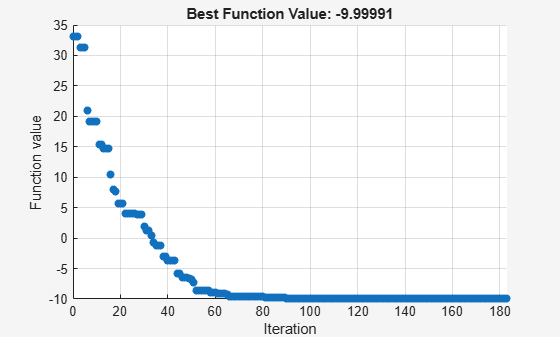# Explore `patternsearch` Algorithms

Starting with R2022b, `patternsearch` has four algorithms:

• `"classic"`

• `"nups"` (Nonuniform Pattern Search)

• `"nups-gps"`

• `"nups-mads"`

This example shows how the choice of algorithm affects a bounded problem with a nonsmooth objective function.

### Objective Function

The objective function for this example is based on the 2-D `ps_example` function that is available when you run the example.

`type ps_example`
```function f = ps_example(x) %PS_EXAMPLE objective function for patternsearch. % Copyright 2003-2021 The MathWorks, Inc. f = zeros(1,size(x,1)); for i = 1:size(x,1) if x(i,1) < -5 f(i) = (x(i,1)+5)^2 + abs(x(i,2)); elseif x(i,1) < -3 f(i) = -2*sin(x(i,1)) + abs(x(i,2)); elseif x(i,1) < 0 f(i) = 0.5*x(i,1) + 2 + abs(x(i,2)); elseif x(i,1) >= 0 f(i) = .3*sqrt(x(i,1)) + 5/2 +abs(x(i,2)); end end ```

Extend the `ps_example` function to any even number of dimensions by creating pseudorandom offsets from the origin for every two dimensions, and adding the resulting objectives. See the code for the `testps` helper function at the end of this example.

Specify `N = 10` dimensions, and set bounds of –20 to 20 for each component. Start `patternsearch` from the point `x0 = [0 0 ... 0]`.

```N = 10; lb = -20*ones(1,N); ub = -lb; x0 = zeros(size(lb));```

### Run Classic Algorithm

Set options to use the `"classic"` algorithm and to display the objective function values.

`optscl = optimoptions("patternsearch",Algorithm="classic",PlotFcn="psplotbestf");`

Run the optimization.

```[xclassic,fvalclassic,eflagclassic,outputclassic] = ... patternsearch(@testps,x0,[],[],[],[],lb,ub,[],optscl)```
```patternsearch stopped because the mesh size was less than options.MeshTolerance. ``````xclassic = 1×10 -7.9575 5.9058 -8.5047 -5.5481 -8.9402 -2.8633 -7.5058 0.8919 -5.6967 2.9322 ```
```fvalclassic = -10.0000 ```
```eflagclassic = 1 ```
```outputclassic = struct with fields: function: @testps problemtype: 'boundconstraints' pollmethod: 'gpspositivebasis2n' maxconstraint: 0 searchmethod: [] iterations: 218 funccount: 3487 meshsize: 9.5367e-07 rngstate: [1x1 struct] message: 'patternsearch stopped because the mesh size was less than options.MeshTolerance.' ```

### Run NUPS Algorithm

Set options to use the `"nups"` algorithm. To obtain reproducible results, set the random seed.

```optsnups = optimoptions(optscl,Algorithm="nups"); rng default % For reproducibility [xnups,fvalnups,eflagnups,outputnups] = ... patternsearch(@testps,x0,[],[],[],[],lb,ub,[],optsnups)```
```patternsearch stopped because the mesh size was less than options.MeshTolerance. ``````xnups = 1×10 -7.9575 5.9058 -8.5047 -5.5480 -8.9401 -2.8633 -7.5058 0.8919 -5.6967 2.9322 ```
```fvalnups = -9.9999 ```
```eflagnups = 1 ```
```outputnups = struct with fields: function: @testps problemtype: 'boundconstraints' pollmethod: 'nups' maxconstraint: 0 searchmethod: [] iterations: 183 funccount: 1827 meshsize: 8.5928e-07 rngstate: [1x1 struct] message: 'patternsearch stopped because the mesh size was less than options.MeshTolerance.' ```

In this case, the `"nups"` algorithm reaches essentially the same solution as the `"classic"` algorithm while using fewer function evaluations.

### Further Explorations

You can also try the `"nups-gps"` and `"nups-mads"` algorithms to see how they perform on this problem. Although you cannot always predict which algorithm works best on a problem, `"nups"` can be a good starting algorithm because it incorporates many adaptive features, making it likely to be the best algorithm.

### Helper Function

This code creates the `testps` helper function.

```function y = testps(x) N = numel(x); % Number of variables if mod(N,2) == 1 disp("Number of variables must be even.") return end strm = RandStream("twister",Seed=1); % Set Seed for consistency % Use RandStream to avoid affecting the global stream dsp = 5*randn(strm,size(x)); z = x - dsp; % Include random offsets y = 0; for i = 1:N/2 y = y + ps_example(z([2*i-1,2*i])); end end```